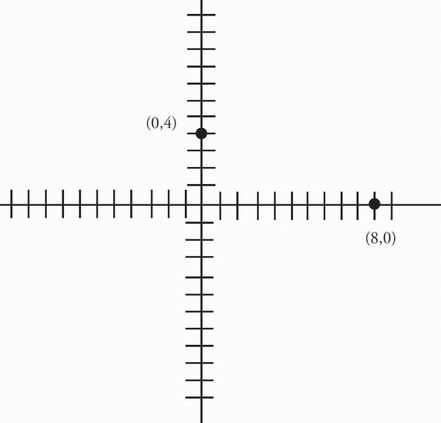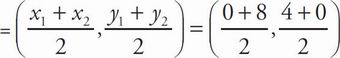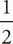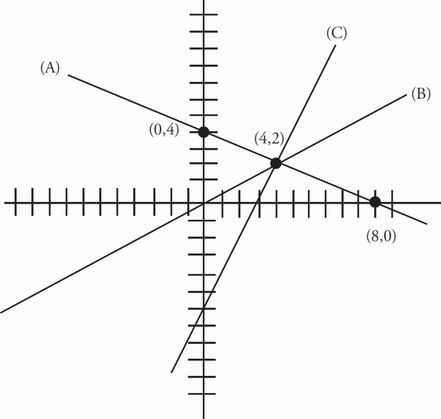# SAT Math Multiple Choice Question 165: Answer and Explanation

### Test Information

Question: 165

15. Which of the following lines contains all points equidistant from the points (0, 4) and (8, 0) in the xy-plane?

• A. 2y = -x + 8
• B. 2y = x
• C. y = 2x - 6
• D. y = -2xThe point directly between the two points will definitely be on the line, so find the midpoint of the two points. Midpoint= (4, 2). Check this point in the answer choices and eliminate any that do not contain it. Choice (A) becomes 2(2) = -4 + 8 or 4 = 4, which is true. Choice (B) becomes 2(2) = 4, and (C) becomes 2 = 2(4) - 6 or 2 = 8 - 6. These are also true, but (D) becomes 2 = -2(4), which is false. Eliminate (D). To sketch the remaining equations, rewrite them in slope-intercept form of the equation y = mx + b, where m is the slope and b is the y-intercept. Choice (A) becomes y = -x + 4, (B) becomes y =x, and (C) is already in the right form. Now sketch the graphs of each of these on the xy-plane.